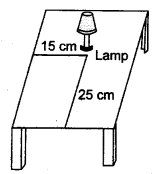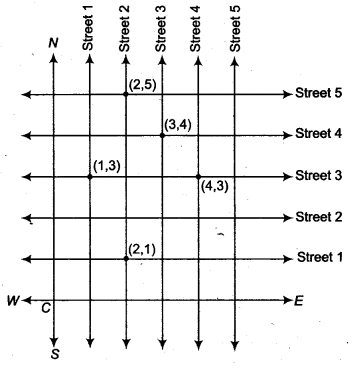## NCERT Solutions for Class 9 Maths Chapter 6 Coordinate Geometry Ex 6.1

NCERT Solutions for Class 9 Maths Chapter 6 Coordinate Geometry Ex 6.1 are part of NCERT Solutions for Class 9 Maths. Here we have given NCERT Solutions for Class 9 Maths Chapter 6 Coordinate Geometry Ex 6.1.

 Board CBSE Textbook NCERT Class Class 9 Subject Maths Chapter Chapter 6 Chapter Name Coordinate Geometry Exercise Ex 6.1 Number of Questions Solved 2 Category NCERT Solutions

## NCERT Solutions for Class 9 Maths Chapter 6 Coordinate Geometry Ex 6.1

Question 1.
How will you describe the position of a table lamp on your study table to another person?
Solution:
Let us consider the lamp as a point A and table as a plane.Take any two perpendicular edges of the table, say OX and OY. Measure the distance of the lamp A from the larger edge OX, let it be 25 cm. Again, measure the distance of the lamp A from the shorter edge OY, let it be 15 cm. Therefore, the position of the lamp A to the edges OX and OY is (15, 25).

Question 2.
(Street Plan) A city has two main roads which cross each other at the centre of the city. These two roads are along the North-South direction and East-West direction. All the other streets of the city run parallel to these roads and are 200 m apart. There are 5 streets in each direction. Using 1 cm = 200 m, draw a model of the city on your notebook. Represent the roads/streets by single lines.
There are many cross-streets in your model. A particular cross-street is made by two street one running in the North-South direction and another in the East-West direction. Each cross-street is referred to in the following manner : If the 2 nd street running in the North-South direction and 5th in the East-West direction meet at some crossing, then we will call this cross-street (2, 5). Using this convention, find
(i) how many cross-streets can be referred to as (4, 3).
(ii) how many cross-streets can be referred to as (3, 4).
Solution:
(i) The street plan is shown by the following figure.(i) There is only one cros.s-street which can be referred as (4, 3).
(ii) There is only one cross-street which can be referred as (3, 4).
We hope the NCERT Solutions for Class 9 Maths Chapter 6 Coordinate Geometry Ex 6.1 help you. If you have any query regarding NCERT Solutions for Class 9 Maths Chapter 6 Coordinate Geometry Ex 6.1, drop a comment below and we will get back to you at the earliest.